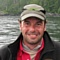Project Management Central

7 Points off the meanNetwork:84I ran across a question in the PMChallenge quiz that aroused my curiosity. It asked how many consecutive points above or below the mean of a process metric indicated that it was out of statistical control. The answer is 7, but it occurred to me that I did not know the statistical basis for this answer. The reference cited is to a chapter in PMBOK v5, which I no longer have access to, so that didn't help, and I doubt that it contained the math anyway. I tried a few google searches, and it is a well-accepted principle in Lean management techniques, but I still couldn't find the underlying math. Can anyone point me to a reference??
Sort By:Network:11Hope this helps, (This is not my work)
Run Chart: A Run Chart simply plots the data of a variable over time.
Through analysis of a run chart, the following can be derived:
changes / trends of the process over time
any pattern / cycle of the process
Examples of a run chart:
progress of the project / processes / tasks (percentage completion over time)
expenditure of the project
Plus: A run chart is easy to draw and interpret. It is useful for analysis of simple processes.
Minus: Cannot show if the process is in control or stable. Not quite useful for quality control.
Control Chart: A Control Chart also plots the data of a variable over time (same as the run chart), but also includes specification limits (Upper Specification Limit USL and Lower Specification Limit LSL) and control limits (Upper Control Limit UCL and Lower Control Limit LCL).
Specification Limits: provided in the project plan / contract as a project requirement of the process;
Control Limits: specified by the quality requirements of the process (e.g. 3-sigma); if a data go beyond the control limits or a pattern/trend has been formed, corrective actions must be taken to correction the deviation.
Tells whether the process is under control
Telltales of “Out of Control”:
Any data point outside the control limits
Rule of 7: 7 consecutive data points within the control limits but on either side of the mean
A trend has been formed (e.g. 6 consecutive points forming an increasing or decreasing trend
From: https://edward-designer.com/web/run-chart-...t-for-pmp-exam/Network:1613Joseph -

it comes down to the probability of repeated variation in one direction of a normal distribution where the mean and median are the same. If we assume that 50% of the time we will be on one side or the other, then getting 7 times on the same side would be .5^6 which is about 1.5% so is low enough to not be random variation.

KironNetwork:333Minitab uses 6, the Nelson rules  use 9 points (from Grant and Leavenworth ). The rule of 7 is the common rule of thumb (heuristic) for when there is an apparent mean shift.

It comes down to the probability that the indicator will give a false positive. At 9 points, the probability is about 1%, at 7 it's 1.6%, at lower numbers you have a higher probability of a false positive.

If you want scholarly articles, search for "probability of mean shift".Network:27174It has to do with how you want to control your process. other parameters of chart or your control plan are important as well. The 7-point rule is one of the first ones introduced many years ago.